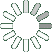Puzzle Command
Daily Puzzle
Number Logic #17
February 22, 2015Find a 6 digit number where:

The 1st digit is odd and the sum of the 2nd and 3rd digit.
The 2nd digit is equal to 2.
The 3rd digit is 0, 1, 2, 3, 4, 5, 6, 7, 8, 9, or 0.
The 4th digit is the sum of the 1st, 2nd, and 3rd digit.
The 5th digit is the sum of the 4th and 6th digit.
The 6th digit is even.

Puzzle Notes
6 digit numbers range from 100,000 to 999,999.
Enter in your solution below and click submit.